International
Tables for
Crystallography
Volume B
Reciprocal space
Edited by U. Shmueli

International Tables for Crystallography (2010). Vol. B, ch. 1.2, pp. 16-17

## Table 1.2.7.3

P. Coppensa*

aDepartment of Chemistry, Natural Sciences & Mathematics Complex, State University of New York at Buffalo, Buffalo, New York 14260–3000, USA
Correspondence e-mail: coppens@buffalo.edu

 Table 1.2.7.3| top | pdf | `Kubic harmonic' functions
 (a) Coefficients in the expression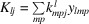with normalization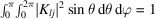(Kara & Kurki-Suonio, 1981).
Even lmp
lj0+2+4+6+8+10+
0 1 1
4 10.76376   0.64550
6 10.35355   −0.93541
6 20.82916   −0.55902
8 10.71807   0.38188   0.58184
10 10.41143   −0.58630   −0.69784
10 20.80202   0.15729   0.57622
l j   2− 4− 6− 8−
3 1   1
7 10.73598   0.41458
9 10.43301   −0.90139
9 20.84163   −0.54006
 (b) Coefficients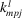and density normalization factorsin the expression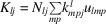where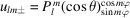(Su & Coppens, 1994).
Even lmp
lj 0+2+4+6+8+10+
0 11
4 1 0.43454 16 1 0.25220 16 2 0.020833   18 1 0.56292 1   1/594010 1 0.36490 1   1/546010 2 0.0095165 1l j     2− 4− 6− 8−
3 1 0.066667   1
7 1 0.014612   19 1 0.0059569   19 2 0.00014800     1(c) Density-normalized Kubic harmonics as linear combinations of density-normalized spherical harmonic functions. Coefficients in the expression. Density-type normalization is defined as.
Even lmp
lj0+2+4+6+8+10+
0 1 1
4 1 0.78245   0.57939
6 1 0.37790   −0.91682
6 2   0.83848   −0.50000
l j 2− 4− 6− 8−
3 1 1
7 1 0.73145   0.63290
 (d) Index rules for cubic symmetries (Kurki-Suonio, 1977; Kara & Kurki-Suonio, 1981).
lj23432TO0 1 × × × × ×
3 1 ×     ×
4 1 × × × × ×
6 1 × × × × ×
6 2 × ×
7 1 ×     ×
8 1 × × × × ×
9 1 ×     ×
9 2 ×   ×
10 1 × × × × ×
10 2 × ×